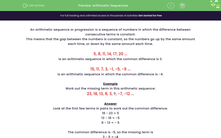# Find the Missing Number in a Sequence

In this worksheet, students will find the missing term in arithmetic sequences.Key stage:  KS 3

Curriculum topic:   Algebra

Curriculum subtopic:   Generate Terms of a Sequence

Popular topics:   Algebra worksheets

Difficulty level:#### Worksheet Overview

An arithmetic sequence or progression is a sequence of numbers in which the difference between consecutive terms is constant.

This means that the gap between the numbers is constant, so the numbers go up by the same amount each time, or down by the same amount each time.

5, 8, 11, 14, 17, 20 ...

is an arithmetic sequence in which the common difference is 3.

15, 11, 7, 3, -1, -5, -9 ...

is an arithmetic sequence in which the common difference is -4.

Example

Work out the missing term in this arithmetic sequence:

23, 18, 13, 8, 3, ?, -7, -12 ...

Look at the first few terms in pairs to work out the common difference.

18 - 23 = -5

13 - 18 = -5

8 - 13 = - 5

The common difference is -5, so the missing term is

3 - 5 = -2

Once you've worked out your missing term, it is always a good idea to check that the sequence works when this term is added to it.

This makes sure that you don't make a silly mistake!Let's get started!

### What is EdPlace?

We're your National Curriculum aligned online education content provider helping each child succeed in English, maths and science from year 1 to GCSE. With an EdPlace account you’ll be able to track and measure progress, helping each child achieve their best. We build confidence and attainment by personalising each child’s learning at a level that suits them.

Get started